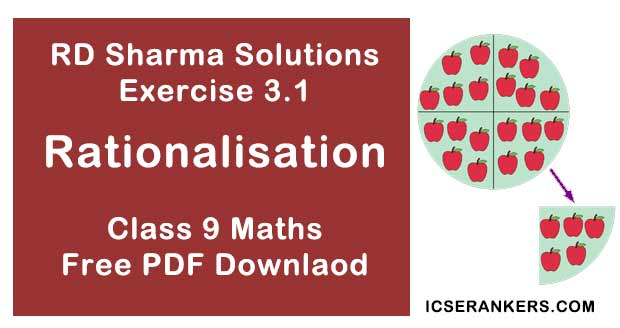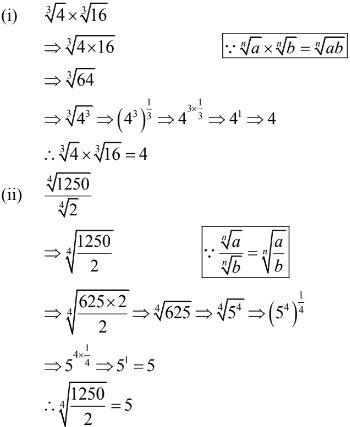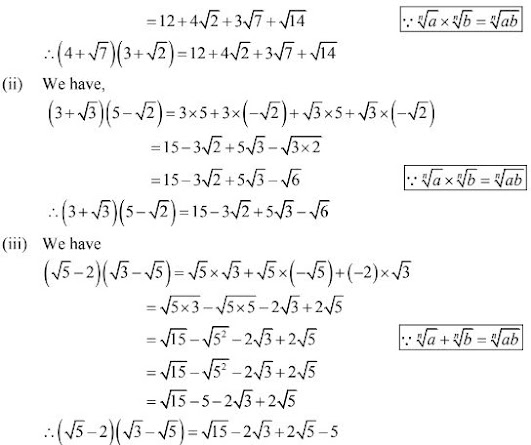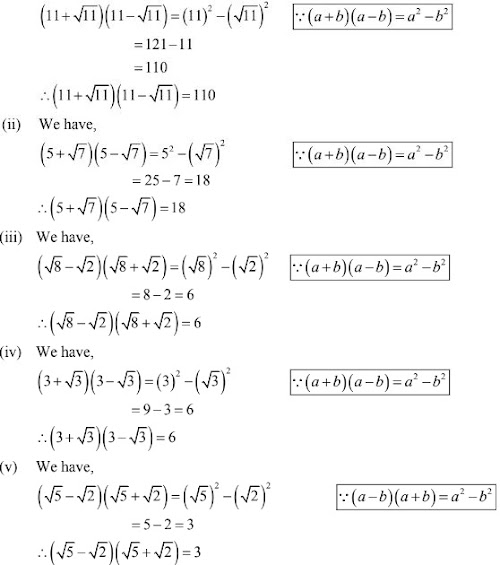# Chapter 3 Rationalisation RD Sharma Solutions Exercise 3.1 Class 9 MathsChapter Name RD Sharma Chapter 3 Rationalisation Exercise 3.1 Book Name RD Sharma Mathematics for Class 10 Other Exercises Exercise 3.2 Related Study NCERT Solutions for Class 10 Maths

### Exercise 3.1 Solutions

1. Simplify each of the following :
(i) ∛4 × ∛16
(ii) ∜1250/∜2

Solution2. Simplify the following expressions :
(i) (4 + √7)(3 + √2)
(ii) (3+ √3)(5 - √2)
(iii) (√5 - 2)(√3 - √5)

Solution

(i) We have
(4 + √7)(3 + √2)
= 4×3+ 4×√2 + √7 × 3 + √7×3 + √7×√2
= 12 + 4√2 + 3√7 + √(7×2)3. Simplify the following expressions :
(i) (11 + √11)(11 - √11)
(ii) (5 + √7)(5 - √7)
(iii) (√8 - √2)(√8 + √2)
(iv) (3 + √3)(3 - √3)
(v) (√5 - √2)(√5 + √2)

Solution

(i) We have,